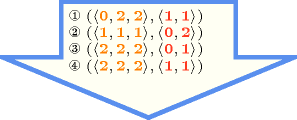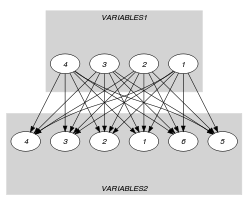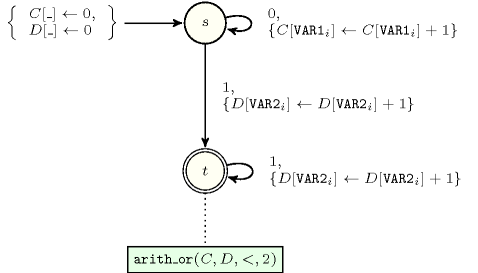## 5.123. disjoint

Origin
Constraint

$\mathrm{𝚍𝚒𝚜𝚓𝚘𝚒𝚗𝚝}\left(\mathrm{𝚅𝙰𝚁𝙸𝙰𝙱𝙻𝙴𝚂}\mathtt{1},\mathrm{𝚅𝙰𝚁𝙸𝙰𝙱𝙻𝙴𝚂}\mathtt{2}\right)$

Arguments
 $\mathrm{𝚅𝙰𝚁𝙸𝙰𝙱𝙻𝙴𝚂}\mathtt{1}$ $\mathrm{𝚌𝚘𝚕𝚕𝚎𝚌𝚝𝚒𝚘𝚗}\left(\mathrm{𝚟𝚊𝚛}-\mathrm{𝚍𝚟𝚊𝚛}\right)$ $\mathrm{𝚅𝙰𝚁𝙸𝙰𝙱𝙻𝙴𝚂}\mathtt{2}$ $\mathrm{𝚌𝚘𝚕𝚕𝚎𝚌𝚝𝚒𝚘𝚗}\left(\mathrm{𝚟𝚊𝚛}-\mathrm{𝚍𝚟𝚊𝚛}\right)$
Restrictions
 $\mathrm{𝚛𝚎𝚚𝚞𝚒𝚛𝚎𝚍}$$\left(\mathrm{𝚅𝙰𝚁𝙸𝙰𝙱𝙻𝙴𝚂}\mathtt{1},\mathrm{𝚟𝚊𝚛}\right)$ $\mathrm{𝚛𝚎𝚚𝚞𝚒𝚛𝚎𝚍}$$\left(\mathrm{𝚅𝙰𝚁𝙸𝙰𝙱𝙻𝙴𝚂}\mathtt{2},\mathrm{𝚟𝚊𝚛}\right)$
Purpose

Each variable of the collection $\mathrm{𝚅𝙰𝚁𝙸𝙰𝙱𝙻𝙴𝚂}\mathtt{1}$ should take a value that is distinct from all the values assigned to the variables of the collection $\mathrm{𝚅𝙰𝚁𝙸𝙰𝙱𝙻𝙴𝚂}\mathtt{2}$.

Example
$\left(〈1,9,1,5〉,〈2,7,7,0,6,8〉\right)$

In this example, values $1,5,9$ are used by the variables of $\mathrm{𝚅𝙰𝚁𝙸𝙰𝙱𝙻𝙴𝚂}\mathtt{1}$ and values $0,2,6,7,8$ by the variables of $\mathrm{𝚅𝙰𝚁𝙸𝙰𝙱𝙻𝙴𝚂}\mathtt{2}$. Since there is no intersection between the two previous sets of values the $\mathrm{𝚍𝚒𝚜𝚓𝚘𝚒𝚗𝚝}$ constraint holds.

All solutions

Figure 5.123.1 gives all solutions to the following non ground instance of the $\mathrm{𝚍𝚒𝚜𝚓𝚘𝚒𝚗𝚝}$ constraint: ${U}_{1}\in \left[0..2\right]$, ${U}_{2}\in \left[1..2\right]$, ${U}_{3}\in \left[1..2\right]$, ${V}_{1}\in \left[0..1\right]$, ${V}_{2}\in \left[1..2\right]$, $\mathrm{𝚍𝚒𝚜𝚓𝚘𝚒𝚗𝚝}$$\left(〈{U}_{1},{U}_{2},{U}_{3}〉,〈{V}_{1},{V}_{2}〉\right)$.

##### Figure 5.123.1. All solutions corresponding to the non ground example of the $\mathrm{𝚍𝚒𝚜𝚓𝚘𝚒𝚗𝚝}$ constraint of the All solutions slotTypical
 $|\mathrm{𝚅𝙰𝚁𝙸𝙰𝙱𝙻𝙴𝚂}\mathtt{1}|>1$ $|\mathrm{𝚅𝙰𝚁𝙸𝙰𝙱𝙻𝙴𝚂}\mathtt{2}|>1$
Symmetries
• Arguments are permutable w.r.t. permutation $\left(\mathrm{𝚅𝙰𝚁𝙸𝙰𝙱𝙻𝙴𝚂}\mathtt{1},\mathrm{𝚅𝙰𝚁𝙸𝙰𝙱𝙻𝙴𝚂}\mathtt{2}\right)$.

• Items of $\mathrm{𝚅𝙰𝚁𝙸𝙰𝙱𝙻𝙴𝚂}\mathtt{1}$ are permutable.

• Items of $\mathrm{𝚅𝙰𝚁𝙸𝙰𝙱𝙻𝙴𝚂}\mathtt{2}$ are permutable.

• An occurrence of a value of $\mathrm{𝚅𝙰𝚁𝙸𝙰𝙱𝙻𝙴𝚂}\mathtt{1}.\mathrm{𝚟𝚊𝚛}$ can be replaced by any value of $\mathrm{𝚅𝙰𝚁𝙸𝙰𝙱𝙻𝙴𝚂}\mathtt{1}.\mathrm{𝚟𝚊𝚛}$.

• An occurrence of a value of $\mathrm{𝚅𝙰𝚁𝙸𝙰𝙱𝙻𝙴𝚂}\mathtt{2}.\mathrm{𝚟𝚊𝚛}$ can be replaced by any value of $\mathrm{𝚅𝙰𝚁𝙸𝙰𝙱𝙻𝙴𝚂}\mathtt{2}.\mathrm{𝚟𝚊𝚛}$.

• All occurrences of two distinct values in $\mathrm{𝚅𝙰𝚁𝙸𝙰𝙱𝙻𝙴𝚂}\mathtt{1}.\mathrm{𝚟𝚊𝚛}$ or $\mathrm{𝚅𝙰𝚁𝙸𝙰𝙱𝙻𝙴𝚂}\mathtt{2}.\mathrm{𝚟𝚊𝚛}$ can be swapped; all occurrences of a value in $\mathrm{𝚅𝙰𝚁𝙸𝙰𝙱𝙻𝙴𝚂}\mathtt{1}.\mathrm{𝚟𝚊𝚛}$ or $\mathrm{𝚅𝙰𝚁𝙸𝙰𝙱𝙻𝙴𝚂}\mathtt{2}.\mathrm{𝚟𝚊𝚛}$ can be renamed to any unused value.

Arg. properties
• Contractible wrt. $\mathrm{𝚅𝙰𝚁𝙸𝙰𝙱𝙻𝙴𝚂}\mathtt{1}$.

• Contractible wrt. $\mathrm{𝚅𝙰𝚁𝙸𝙰𝙱𝙻𝙴𝚂}\mathtt{2}$.

Remark

Despite the fact that this is not an uncommon constraint, it can not be modelled in a compact way neither with a disequality constraint (i.e., two given variables have to take distinct values) nor with the $\mathrm{𝚊𝚕𝚕𝚍𝚒𝚏𝚏𝚎𝚛𝚎𝚗𝚝}$ constraint. The $\mathrm{𝚍𝚒𝚜𝚓𝚘𝚒𝚗𝚝}$ constraint can bee seen as a special case of the $\mathrm{𝚌𝚘𝚖𝚖𝚘𝚗}$$\left(\mathrm{𝙽𝙲𝙾𝙼𝙼𝙾𝙽}\mathtt{1},\mathrm{𝙽𝙲𝙾𝙼𝙼𝙾𝙽}\mathtt{2},\mathrm{𝚅𝙰𝚁𝙸𝙰𝙱𝙻𝙴𝚂}\mathtt{1},\mathrm{𝚅𝙰𝚁𝙸𝙰𝙱𝙻𝙴𝚂}\mathtt{2}\right)$ constraint where $\mathrm{𝙽𝙲𝙾𝙼𝙼𝙾𝙽}\mathtt{1}$ and $\mathrm{𝙽𝙲𝙾𝙼𝙼𝙾𝙽}\mathtt{2}$ are both set to 0.

MiniZinc (http://www.minizinc.org/) has a $\mathrm{𝚍𝚒𝚜𝚓𝚘𝚒𝚗𝚝}$ constraint between two set variables rather than between two collections of variables.

Algorithm

Let us note:

• ${n}_{1}$ the minimum number of distinct values taken by the variables of the collection $\mathrm{𝚅𝙰𝚁𝙸𝙰𝙱𝙻𝙴𝚂}\mathtt{1}$.

• ${n}_{2}$ the minimum number of distinct values taken by the variables of the collection $\mathrm{𝚅𝙰𝚁𝙸𝙰𝙱𝙻𝙴𝚂}\mathtt{2}$.

• ${n}_{12}$ the maximum number of distinct values taken by the union of the variables of $\mathrm{𝚅𝙰𝚁𝙸𝙰𝙱𝙻𝙴𝚂}\mathtt{1}$ and $\mathrm{𝚅𝙰𝚁𝙸𝙰𝙱𝙻𝙴𝚂}\mathtt{2}$.

One invariant to maintain for the $\mathrm{𝚍𝚒𝚜𝚓𝚘𝚒𝚗𝚝}$ constraint is ${n}_{1}+{n}_{2}\le {n}_{12}$. A lower bound of ${n}_{1}$ and ${n}_{2}$ can be obtained by using the algorithms provided in [Beldiceanu01], [BeldiceanuCarlssonThiel02]. An exact upper bound of ${n}_{12}$ can be computed by using a bipartite matching algorithm.

Used in

generalisation: $\mathrm{𝚍𝚒𝚜𝚓𝚘𝚒𝚗𝚝}_\mathrm{𝚝𝚊𝚜𝚔𝚜}$ ($\mathrm{𝚟𝚊𝚛𝚒𝚊𝚋𝚕𝚎}$ replaced by $\mathrm{𝚝𝚊𝚜𝚔}$).

Keywords
Arc input(s)

$\mathrm{𝚅𝙰𝚁𝙸𝙰𝙱𝙻𝙴𝚂}\mathtt{1}$ $\mathrm{𝚅𝙰𝚁𝙸𝙰𝙱𝙻𝙴𝚂}\mathtt{2}$

Arc generator
$\mathrm{𝑃𝑅𝑂𝐷𝑈𝐶𝑇}$$↦\mathrm{𝚌𝚘𝚕𝚕𝚎𝚌𝚝𝚒𝚘𝚗}\left(\mathrm{𝚟𝚊𝚛𝚒𝚊𝚋𝚕𝚎𝚜}\mathtt{1},\mathrm{𝚟𝚊𝚛𝚒𝚊𝚋𝚕𝚎𝚜}\mathtt{2}\right)$

Arc arity
Arc constraint(s)
$\mathrm{𝚟𝚊𝚛𝚒𝚊𝚋𝚕𝚎𝚜}\mathtt{1}.\mathrm{𝚟𝚊𝚛}=\mathrm{𝚟𝚊𝚛𝚒𝚊𝚋𝚕𝚎𝚜}\mathtt{2}.\mathrm{𝚟𝚊𝚛}$
Graph property(ies)
$\mathrm{𝐍𝐀𝐑𝐂}$$=0$

Graph model

$\mathrm{𝑃𝑅𝑂𝐷𝑈𝐶𝑇}$ is used in order to generate the arcs of the graph between all variables of $\mathrm{𝚅𝙰𝚁𝙸𝙰𝙱𝙻𝙴𝚂}\mathtt{1}$ and all variables of $\mathrm{𝚅𝙰𝚁𝙸𝙰𝙱𝙻𝙴𝚂}\mathtt{2}$. Since we use the graph property $\mathrm{𝐍𝐀𝐑𝐂}$ $=$ 0 the final graph will be empty. Figure 5.123.2 shows the initial graph associated with the Example slot. Since we use the $\mathrm{𝐍𝐀𝐑𝐂}$ $=$ 0 graph property the final graph is empty.

##### Figure 5.123.2. Initial graph of the $\mathrm{𝚍𝚒𝚜𝚓𝚘𝚒𝚗𝚝}$ constraint (the final graph is empty)Signature

Since 0 is the smallest number of arcs of the final graph we can rewrite $\mathrm{𝐍𝐀𝐑𝐂}$ $=$ 0 to $\mathrm{𝐍𝐀𝐑𝐂}$ $\le$ 0. This leads to simplify $\underline{\overline{\mathrm{𝐍𝐀𝐑𝐂}}}$ to $\underline{\mathrm{𝐍𝐀𝐑𝐂}}$.

Automaton

Figure 5.123.3 depicts the automaton associated with the $\mathrm{𝚍𝚒𝚜𝚓𝚘𝚒𝚗𝚝}$ constraint. To each variable $\mathrm{𝚅𝙰𝚁}{\mathtt{1}}_{i}$ of the collection $\mathrm{𝚅𝙰𝚁𝙸𝙰𝙱𝙻𝙴𝚂}\mathtt{1}$ corresponds a signature variable ${S}_{i}$ that is equal to 0. To each variable $\mathrm{𝚅𝙰𝚁}{\mathtt{2}}_{i}$ of the collection $\mathrm{𝚅𝙰𝚁𝙸𝙰𝙱𝙻𝙴𝚂}\mathtt{2}$ corresponds a signature variable ${S}_{i+|\mathrm{𝚅𝙰𝚁𝙸𝙰𝙱𝙻𝙴𝚂}\mathtt{1}|}$ that is equal to 1.

##### Figure 5.123.3. Automaton of the $\mathrm{𝚍𝚒𝚜𝚓𝚘𝚒𝚗𝚝}$$\left(\mathrm{𝚅𝙰𝚁𝙸𝙰𝙱𝙻𝙴𝚂}\mathtt{1},\mathrm{𝚅𝙰𝚁𝙸𝙰𝙱𝙻𝙴𝚂}\mathtt{2}\right)$ constraint, where state $s$ handles variables of the collection $\mathrm{𝚅𝙰𝚁𝙸𝙰𝙱𝙻𝙴𝚂}\mathtt{1}$ and state $t$ handles variables of the collection $\mathrm{𝚅𝙰𝚁𝙸𝙰𝙱𝙻𝙴𝚂}\mathtt{2}$